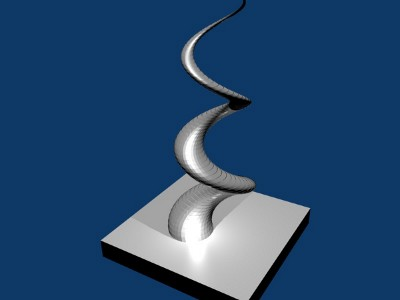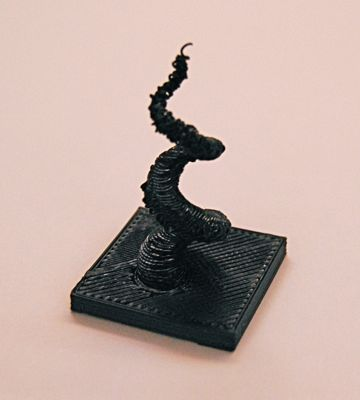## Printed Processing sketch

I just printed the first stl file that i generated using a processing sketch.

I used the unlekkerlib to export a stl file from a sketch that generates simple 3d spiral, used blender to add a socket and printed it on my makerbot. Skeinforge complained about some invalid triangles, but beside that it worked surprisingly well.

This is what it looks like in blenderand this is what the makerbot made of itand this is the processing-sketch i used to generated the spiral

``````import unlekker.data.*;

void setup() {
size(300,300,P3D);
noLoop();
}

void draw() {
translate(width/2,height/2);
background(0);
fill(255);
lights();
noStroke();
//stroke(255);
beginRaw("unlekker.data.STL","guru.stl");
for( int i =0; i < 100; i++ ) {
for(int a=0; a < 36; a++) {
float r = 10 - map(i,0,100,0,10);
vertex( r * sin( radians( a * 10 )) + sin(radians(i*10)) * 10,
-i*1,

int j = i+1;
vertex( r * sin( radians( a * 10 )) + sin(radians(j*10)) * 10,
-j*1,
}
}
endShape();
endRaw();
}
``````